Technical Test SSC JE: Electrical Engineering (EE)- 1

# Technical Test SSC JE: Electrical Engineering (EE)- 1

Test Description

## 100 Questions MCQ Test Mock Test Series for SSC JE Electrical Engineering | Technical Test SSC JE: Electrical Engineering (EE)- 1

Technical Test SSC JE: Electrical Engineering (EE)- 1 for Electrical Engineering (EE) 2022 is part of Mock Test Series for SSC JE Electrical Engineering preparation. The Technical Test SSC JE: Electrical Engineering (EE)- 1 questions and answers have been prepared according to the Electrical Engineering (EE) exam syllabus.The Technical Test SSC JE: Electrical Engineering (EE)- 1 MCQs are made for Electrical Engineering (EE) 2022 Exam. Find important definitions, questions, notes, meanings, examples, exercises, MCQs and online tests for Technical Test SSC JE: Electrical Engineering (EE)- 1 below.
Solutions of Technical Test SSC JE: Electrical Engineering (EE)- 1 questions in English are available as part of our Mock Test Series for SSC JE Electrical Engineering for Electrical Engineering (EE) & Technical Test SSC JE: Electrical Engineering (EE)- 1 solutions in Hindi for Mock Test Series for SSC JE Electrical Engineering course. Download more important topics, notes, lectures and mock test series for Electrical Engineering (EE) Exam by signing up for free. Attempt Technical Test SSC JE: Electrical Engineering (EE)- 1 | 100 questions in 100 minutes | Mock test for Electrical Engineering (EE) preparation | Free important questions MCQ to study Mock Test Series for SSC JE Electrical Engineering for Electrical Engineering (EE) Exam | Download free PDF with solutions
 1 Crore+ students have signed up on EduRev. Have you?
Technical Test SSC JE: Electrical Engineering (EE)- 1 - Question 1

### Kirchhoff's second law is based on the law of conservation of

Detailed Solution for Technical Test SSC JE: Electrical Engineering (EE)- 1 - Question 1

This law is based on the conservation of energy whereby voltage is defined as the energy per unit charge. The total amount of energy gained per unit charge must be equal to the amount of energy lost per unit charge, as energy and charge are both conserved.

Technical Test SSC JE: Electrical Engineering (EE)- 1 - Question 2

### The resistance temperature coefficient is defined as

Technical Test SSC JE: Electrical Engineering (EE)- 1 - Question 3

### For the circuit shown the reading in the ammeter A will be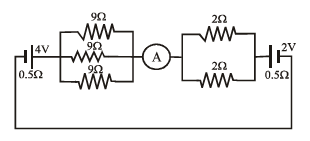Detailed Solution for Technical Test SSC JE: Electrical Engineering (EE)- 1 - Question 3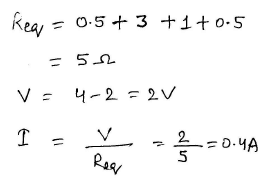Technical Test SSC JE: Electrical Engineering (EE)- 1 - Question 4

When checked with an ohm meter an open resistor reads

Detailed Solution for Technical Test SSC JE: Electrical Engineering (EE)- 1 - Question 4

If you don't connect the probes to anything, you have an open circuit, there is no path from one to the other (except through the air), and the meter will read infinity ohms.

Technical Test SSC JE: Electrical Engineering (EE)- 1 - Question 5

A light dependent resistor is basically a

Detailed Solution for Technical Test SSC JE: Electrical Engineering (EE)- 1 - Question 5

A light dependent resistor abbreviated as LDR is an electronic device which senses light. It is basically a variable resistor whose value varies with the intensity of incident light. It consists of a pair of metal contacts between which a curved track of cadmium sulphide is placed

Technical Test SSC JE: Electrical Engineering (EE)- 1 - Question 6

Voltage dependent resistors are usually made from

Technical Test SSC JE: Electrical Engineering (EE)- 1 - Question 7

Resistors across A & B in the circuit shown below is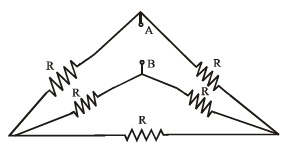Detailed Solution for Technical Test SSC JE: Electrical Engineering (EE)- 1 - Question 7

On rearranging, we get a balanced wheatstone bridge. hence the effective resistance is R

Technical Test SSC JE: Electrical Engineering (EE)- 1 - Question 8

Voltage dependent resistors are used

Detailed Solution for Technical Test SSC JE: Electrical Engineering (EE)- 1 - Question 8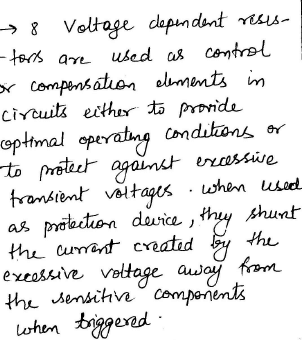Technical Test SSC JE: Electrical Engineering (EE)- 1 - Question 9

The power factor of incandescent bulb is

Detailed Solution for Technical Test SSC JE: Electrical Engineering (EE)- 1 - Question 9

In incandescent bulb there is filament, which is purely restive in nature. and we know that purely resistive element has unity power factor.

Technical Test SSC JE: Electrical Engineering (EE)- 1 - Question 10

The equivalent inductance at seen at terminal AB is_____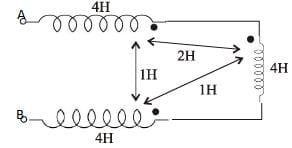Technical Test SSC JE: Electrical Engineering (EE)- 1 - Question 11

If V2 = 1 – e–2t, the value of V1 is given by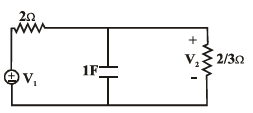Detailed Solution for Technical Test SSC JE: Electrical Engineering (EE)- 1 - Question 11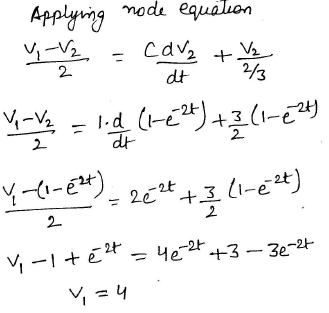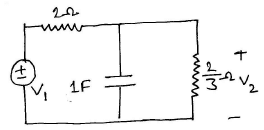Technical Test SSC JE: Electrical Engineering (EE)- 1 - Question 12

For a parallel RLC circuit to be overdamped

Detailed Solution for Technical Test SSC JE: Electrical Engineering (EE)- 1 - Question 12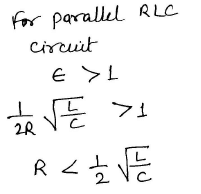Technical Test SSC JE: Electrical Engineering (EE)- 1 - Question 13

The thevenin resistance across the diode in the circuit is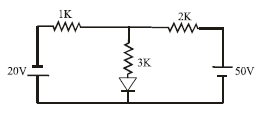Detailed Solution for Technical Test SSC JE: Electrical Engineering (EE)- 1 - Question 13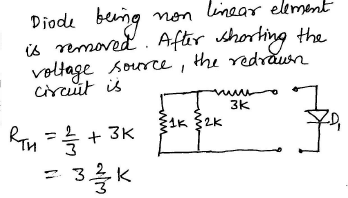Technical Test SSC JE: Electrical Engineering (EE)- 1 - Question 14

Find input resistance of the circuit shown is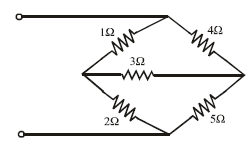Detailed Solution for Technical Test SSC JE: Electrical Engineering (EE)- 1 - Question 14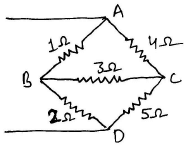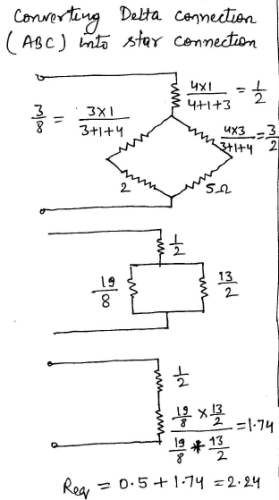Technical Test SSC JE: Electrical Engineering (EE)- 1 - Question 15

A 3H – Inductor has 2000 turns. How many turns must be added to increase the inductance to 5H

Detailed Solution for Technical Test SSC JE: Electrical Engineering (EE)- 1 - Question 15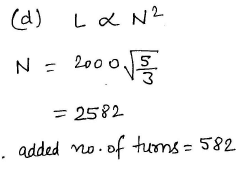Technical Test SSC JE: Electrical Engineering (EE)- 1 - Question 16

For a series RLC circuit the power factor at the lowest half power frequency is

Technical Test SSC JE: Electrical Engineering (EE)- 1 - Question 17

A series RLC circuit resonance at 1MHz at frequency of 1.1 MHz the circuit impedance is

Technical Test SSC JE: Electrical Engineering (EE)- 1 - Question 18

If each branch of a delta circuit has impedance √3z , then each branch of equivalent Y–circuit
has impedance

Detailed Solution for Technical Test SSC JE: Electrical Engineering (EE)- 1 - Question 18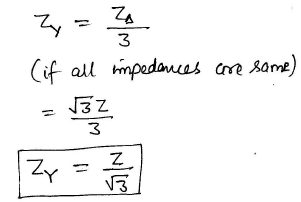Technical Test SSC JE: Electrical Engineering (EE)- 1 - Question 19

A delta load is connected to a balanced 400 V, 3-f supply as shown in figure. The total power dissipated in the network is equal to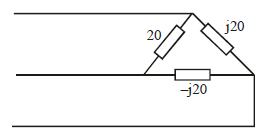Detailed Solution for Technical Test SSC JE: Electrical Engineering (EE)- 1 - Question 19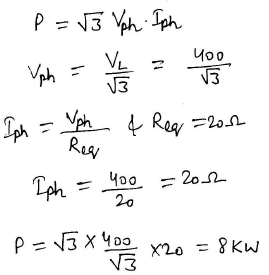Technical Test SSC JE: Electrical Engineering (EE)- 1 - Question 20

When Q-factor of the circuit is high, then

Technical Test SSC JE: Electrical Engineering (EE)- 1 - Question 21

The dynamic resistance of a parallel resonance circuit is given by

Technical Test SSC JE: Electrical Engineering (EE)- 1 - Question 22

A parallel resonant circuit can be employed

Technical Test SSC JE: Electrical Engineering (EE)- 1 - Question 23

A 3-f star connected symmetrical load consumes p watts of power from a balancedsupply. If the same load is connected in delta to the same supply, the power consumption will be

Technical Test SSC JE: Electrical Engineering (EE)- 1 - Question 24

In two wattmeters method of power measurement, one of the wattmeters will show negative reading when the load power factor angle is strictly

Detailed Solution for Technical Test SSC JE: Electrical Engineering (EE)- 1 - Question 24

The reading of a wattmeter is proportional to (current through the current coil)x(voltage across the pressure coil)xCos(angle between this current and this voltage).

So if this angle becomes more than 90 degrees the the reading of the wattmeter will be negative.This could happen in a 3-ph unbalanced circuit.

For a 3-ph balanced load the two wattmeter readings will be,say Vab*Ia*cos (30+phi) and Vcb*Ic* cos(30-phi). So if phi >60 degrees (pf less than 0.5) a negative reading is obtained.

Technical Test SSC JE: Electrical Engineering (EE)- 1 - Question 25

The external characteristics of a shunt generator can be obtained directly from its ____characteristics

Technical Test SSC JE: Electrical Engineering (EE)- 1 - Question 26

For the voltage build up of a self excited d.c. generator, which of the following is not anessential condition?

Technical Test SSC JE: Electrical Engineering (EE)- 1 - Question 27

Which of the following d.c. generator cannot build up on open-circuit?

Technical Test SSC JE: Electrical Engineering (EE)- 1 - Question 28

Which of the following methods of speed control of D.C. machine will offer minimum efficiency?

Technical Test SSC JE: Electrical Engineering (EE)- 1 - Question 29

Which winding of the transformer has less crosssectional area?

Detailed Solution for Technical Test SSC JE: Electrical Engineering (EE)- 1 - Question 29

High voltage winding because coil thickness is low comparing to others

Technical Test SSC JE: Electrical Engineering (EE)- 1 - Question 30

A dc series motor of resistance 1Ω across terminal runs at 1000 rpm at 250V taking acurrent of 20A when an additional resistance 6W is inserted in series and taking the samecurrent, the new speed will be

Detailed Solution for Technical Test SSC JE: Electrical Engineering (EE)- 1 - Question 30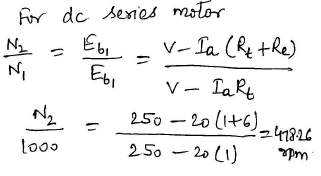Technical Test SSC JE: Electrical Engineering (EE)- 1 - Question 31

In dc motors, the condition for maximum power is

Detailed Solution for Technical Test SSC JE: Electrical Engineering (EE)- 1 - Question 31

The condition for maximum power in case of D.C. motor is

Supply voltage =I × back e.m.f. D.

Technical Test SSC JE: Electrical Engineering (EE)- 1 - Question 32

The speed of a series wound dc motor

Technical Test SSC JE: Electrical Engineering (EE)- 1 - Question 33

In dc series motor 1 shaft torque is less than the armature torque due to

Technical Test SSC JE: Electrical Engineering (EE)- 1 - Question 34

A dc shunt motor has rated rpm of 480 contain industrial application require this motor to runat 540 rpm for sometime which speed control will be desirable

Technical Test SSC JE: Electrical Engineering (EE)- 1 - Question 35

The leakage flux in a transformer depends upon

Technical Test SSC JE: Electrical Engineering (EE)- 1 - Question 36

In a transformer if peak voltage is fed to primary

Technical Test SSC JE: Electrical Engineering (EE)- 1 - Question 37

The phase difference between the primary & secondary voltage of a transformer is

Detailed Solution for Technical Test SSC JE: Electrical Engineering (EE)- 1 - Question 37

There is 90 degree phase shift between voltage and magnetic field. So primary voltage causes 90 degree phase shifted magnetic field, which induces again 90 degree phase shifted secondary voltage. So it adds up to 180 degree. If you change winding direction, secondary voltage will now cancel that phase shift and you will get 0 degree phase shift.

Technical Test SSC JE: Electrical Engineering (EE)- 1 - Question 38

All of the following methods/techniques can be used for the measurement of high ac voltages EXCEPT

Technical Test SSC JE: Electrical Engineering (EE)- 1 - Question 39

Consider the circuit shown in the given figure for maximum power transfer to the load, the primary to secondary turn ratio must be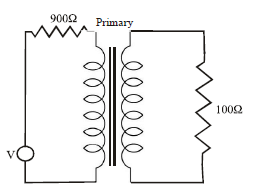Technical Test SSC JE: Electrical Engineering (EE)- 1 - Question 40

Maximum electric power output of a synchronous generator is

Detailed Solution for Technical Test SSC JE: Electrical Engineering (EE)- 1 - Question 40

The power developed in cylindrical synchronous machine is Maximum power developed Pmax = EV/Xs Where E = Excitation voltage V = Terminal voltage Xs = Synchronous reactance δ = load angle Therefore, maximum power developed in cylindrical synchronous machine is at a load angle of 90°.

Technical Test SSC JE: Electrical Engineering (EE)- 1 - Question 41

Two alternators are running in parallel. If the field of one of the alternator is adjusted, it will

Detailed Solution for Technical Test SSC JE: Electrical Engineering (EE)- 1 - Question 41

If the field excitation (voltage adjustment control)of one of a pair of paralleled alternators is turned down, two things will happen: first, the generator in question will develop a less lagging power factor, while the other generator will develop a more lagging power factor, with the overall power factor of the plant and load remaining the same; second, bus voltage, and by extension grid voltage, will drop slightly, unless the other generator’s voltage adjustment (field excitation) is increased proportionally, which will also result in the second generator develop an even more lagging power factor as the first generator’s power factor becomes less lagging.

Technical Test SSC JE: Electrical Engineering (EE)- 1 - Question 42

An unexcited single phase synchronous motor is

Technical Test SSC JE: Electrical Engineering (EE)- 1 - Question 43

A synchronous motor is switched on to supply with its field winding shorted on themselves it will

Technical Test SSC JE: Electrical Engineering (EE)- 1 - Question 44

The power factor of pure resistive circuit is

Technical Test SSC JE: Electrical Engineering (EE)- 1 - Question 45

Under which method of starting an induction motor is expected to take largest starting current?

Detailed Solution for Technical Test SSC JE: Electrical Engineering (EE)- 1 - Question 45

In Direct on line starting, an induction motor is expected to take largest starting current.

In Direct on line starting (DOL) , the contactor will be controlled by separate start and stop buttons, and an auxiliary contact on the contactor is used, across the start button, as a hold in contact. i.e. the contactor is electrically latched closed while the motor is operating.

Technical Test SSC JE: Electrical Engineering (EE)- 1 - Question 46

A dc motors develops a torque of 200 N-m at 25 rps. At 20 rps, it will develop a torque of____ N-m

Detailed Solution for Technical Test SSC JE: Electrical Engineering (EE)- 1 - Question 46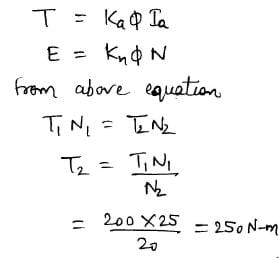Technical Test SSC JE: Electrical Engineering (EE)- 1 - Question 47

An eight pole wound rotor induction motor operating on 60 Hz supply is driven at 1800rpm, by a prime mover in the opposite direction of revolving magnetic field. The frequency ofrotor current is

Detailed Solution for Technical Test SSC JE: Electrical Engineering (EE)- 1 - Question 47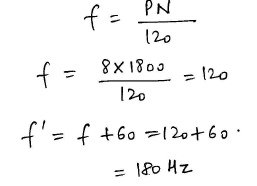Technical Test SSC JE: Electrical Engineering (EE)- 1 - Question 48

A 6-pole, 50 Hz, 3-f induction motor is running at 950 rpm and has rotor Cu loss of 5kw. Its rotor input is ____ kw

Detailed Solution for Technical Test SSC JE: Electrical Engineering (EE)- 1 - Question 48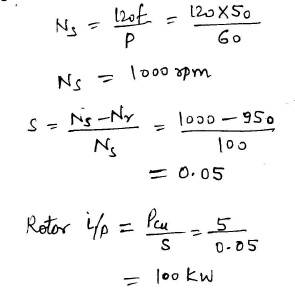Technical Test SSC JE: Electrical Engineering (EE)- 1 - Question 49

A 6 pole, 50 hz-, 3-f induction motor has a full load speed of 950 rpm. At half load, its speed would be ____ rpm

Detailed Solution for Technical Test SSC JE: Electrical Engineering (EE)- 1 - Question 49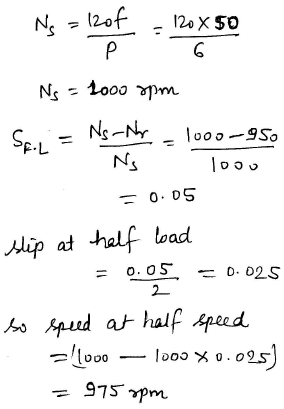Technical Test SSC JE: Electrical Engineering (EE)- 1 - Question 50

A 60 w lamp given a luminous flux of 1500 lumen. Its efficiency is

Technical Test SSC JE: Electrical Engineering (EE)- 1 - Question 51

Which of the following is a cold cathode lamps?

Technical Test SSC JE: Electrical Engineering (EE)- 1 - Question 52

Which of the following lamp has least capacity to sustain voltage fluctuations?

Detailed Solution for Technical Test SSC JE: Electrical Engineering (EE)- 1 - Question 52

Effect of Voltage variation in incandescent lamp

Within the normal range of supply voltage variations the light output will change by about 3.5% for a 1% change of voltage. The effect of voltage on life is much more pronounced; 5% over-voltage will roughly halve the lamp life, whereas 5% under-voltage will approximately double it.

Technical Test SSC JE: Electrical Engineering (EE)- 1 - Question 53

Unbalanced forces are maximum in case of

Technical Test SSC JE: Electrical Engineering (EE)- 1 - Question 54

Which motor is used in tramways

Detailed Solution for Technical Test SSC JE: Electrical Engineering (EE)- 1 - Question 54

Mostly DC series motors are used in this system. For trolley buses and tramways, DC compound motors are used where regenerative braking is required. The various operating voltages of DC traction system include 600V, 750 V, 1500V and 3000V.

Technical Test SSC JE: Electrical Engineering (EE)- 1 - Question 55

In arc welding, the voltage on ac supply system is in the range

Technical Test SSC JE: Electrical Engineering (EE)- 1 - Question 56

In gas welding the gases used are

Detailed Solution for Technical Test SSC JE: Electrical Engineering (EE)- 1 - Question 56

Gases used in welding and cutting processes include: shielding gases such as carbon dioxide, argon, helium, etc. fuel gases such as acetylene, propane, butane, etc. oxygen, used with fuel gases and also in small amounts in some shielding gas mixtures.

Technical Test SSC JE: Electrical Engineering (EE)- 1 - Question 57

For welding duty, the rectifier commonly used are

Detailed Solution for Technical Test SSC JE: Electrical Engineering (EE)- 1 - Question 57

The rectifier types are commonly called "Welding Rectifiers" and produce DC or, AC and DC welding current. They may utilize either single phase or three phase input power. They contain a transformer, but rectify the AC or DC by the use of selenium rectifiers, silicon diodes or silicon controlled rectifiers.

Technical Test SSC JE: Electrical Engineering (EE)- 1 - Question 58

In hygrometers the principle of measurement is

Detailed Solution for Technical Test SSC JE: Electrical Engineering (EE)- 1 - Question 58

Hygrometer uses for measuring the humidity present in the surrounding environment. The term humidity means the amount of water vapour present in the gas. The physical properties of the material changes by the effect of the humidity and this principle use in hygrometer for measurement.

Technical Test SSC JE: Electrical Engineering (EE)- 1 - Question 59

A signal of 10 mv at 75 MHz is to be measured which of the following instrument can be used?

Detailed Solution for Technical Test SSC JE: Electrical Engineering (EE)- 1 - Question 59

At high frequencies, cathode ray oscilloscope  is used

Technical Test SSC JE: Electrical Engineering (EE)- 1 - Question 60

Holes are filled on the opposite side of the disc of an induction type energy meter to

Technical Test SSC JE: Electrical Engineering (EE)- 1 - Question 61

Galvanometer type recorders use

Detailed Solution for Technical Test SSC JE: Electrical Engineering (EE)- 1 - Question 61

In a galvanometer-type recorder, the pointer of the D'Arsonval movement is fitted with a pen-ink (stylus) mechanism. The pointer deflects when current flows through the moving coil.

Technical Test SSC JE: Electrical Engineering (EE)- 1 - Question 62

The curve representing Ohm’s law is

Detailed Solution for Technical Test SSC JE: Electrical Engineering (EE)- 1 - Question 62

From Ohm’s law
V/I = K = R
V = IR
= Linear

Technical Test SSC JE: Electrical Engineering (EE)- 1 - Question 63

A slide wire potentiometer is used to measure voltage across a dc circuit. The voltage is 1.2V as read by the potentiometer. Across the same points 20000 W/V voltmeter reads 0.6V on its 5V range. Input resistance between the two point is

Detailed Solution for Technical Test SSC JE: Electrical Engineering (EE)- 1 - Question 63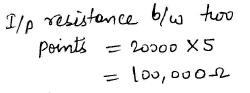Technical Test SSC JE: Electrical Engineering (EE)- 1 - Question 64

In a gravity controlled instrument, the deflection angle is proportional to

Technical Test SSC JE: Electrical Engineering (EE)- 1 - Question 65

In a Q-meter measurement to determine the self capacitance of a coil, the first resonance occurred at F1 with C1 = 30 PF. The second resonance occurred at F2 = 2F1 with C2 = 60PF. The self capacitance of coil works out to be

Technical Test SSC JE: Electrical Engineering (EE)- 1 - Question 66

The deflection of hot wire instrument depends on

Detailed Solution for Technical Test SSC JE: Electrical Engineering (EE)- 1 - Question 66

The deflection of hot wire instrument depends on RMS value of alternating current.

Technical Test SSC JE: Electrical Engineering (EE)- 1 - Question 67

Which one of the following types of instruments can be used to determine the rms value of a.c.voltage of high magnitude (10 KV) and of any wave shape?

Detailed Solution for Technical Test SSC JE: Electrical Engineering (EE)- 1 - Question 67

Electrostatic Instruments are used for voltage measurements only

Technical Test SSC JE: Electrical Engineering (EE)- 1 - Question 68

Eddy current damping is not used on type instruments because

Technical Test SSC JE: Electrical Engineering (EE)- 1 - Question 69

Least expensive instrument for dc measurement is

Technical Test SSC JE: Electrical Engineering (EE)- 1 - Question 70

A Meggar is basically a

Technical Test SSC JE: Electrical Engineering (EE)- 1 - Question 71

The economic size of a conductor is determined by

Detailed Solution for Technical Test SSC JE: Electrical Engineering (EE)- 1 - Question 71

"The Kelvin's law states that the most economical size of a conductor is that for which annual interest and depreciation on the capital cost of the conductor is equal to the annual cost of energy loss."

Technical Test SSC JE: Electrical Engineering (EE)- 1 - Question 72

For a good voltage profile under load condition a long line needs

Detailed Solution for Technical Test SSC JE: Electrical Engineering (EE)- 1 - Question 72

Shunt capacitive compensation. This method is used to improve the power factor. Whenever an inductive load is connected to the transmission line, power factor lags because of lagging load current. To compensate, a shunt capacitor is connected which draws current leading the source voltage.

Technical Test SSC JE: Electrical Engineering (EE)- 1 - Question 73

An industrial consumer has a daily load pattern of 2000 kw, 0.8 p.f. for 12 Hrs and 1000 kw UPF 12 Hrs. The load factor is

Detailed Solution for Technical Test SSC JE: Electrical Engineering (EE)- 1 - Question 73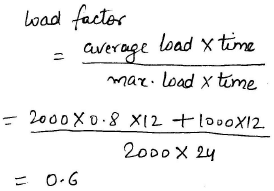Technical Test SSC JE: Electrical Engineering (EE)- 1 - Question 74

The positive sequence reactance will be equal to  the negative sequence reactance.. the equipment is

Technical Test SSC JE: Electrical Engineering (EE)- 1 - Question 75

In overhead transmission lines the effect of capacitance can be neglected when the length of line is less than

Technical Test SSC JE: Electrical Engineering (EE)- 1 - Question 76

Sparking occurs when a load is switched off because the circuit has

Detailed Solution for Technical Test SSC JE: Electrical Engineering (EE)- 1 - Question 76

Wires have inductance associated with it. When load is disconnected current through the wire changes instantaneously. Now inductors can not allow a sudden change in current through them. As a result high voltage grows at the open end of the wire causes breakdown in air. For this there is sparking. Spark plug operates under same principle. Although value of inductance is higher.

Technical Test SSC JE: Electrical Engineering (EE)- 1 - Question 77

Which relay is used for feeders?

Technical Test SSC JE: Electrical Engineering (EE)- 1 - Question 78

Which of the following is a conducting medium for electric current?

Technical Test SSC JE: Electrical Engineering (EE)- 1 - Question 79

Dielectric strength of SF6 is

Detailed Solution for Technical Test SSC JE: Electrical Engineering (EE)- 1 - Question 79

Explanation : The dielectric strength which is 30 % less than that of oil at atmospheric pressure increases rapidly with increase of pressure.

Technical Test SSC JE: Electrical Engineering (EE)- 1 - Question 80

Thermal relays are often used in

Technical Test SSC JE: Electrical Engineering (EE)- 1 - Question 81

Differential protection principle is used in the protection of

Detailed Solution for Technical Test SSC JE: Electrical Engineering (EE)- 1 - Question 81

Differential relay is very commonly used relay for protecting transformers and generators from localised faults. Differential relays are very sensitive to the faults occurred within the zone of protection but they are least sensitive to the faults that occur outside the protected zone.

Technical Test SSC JE: Electrical Engineering (EE)- 1 - Question 82

A cable carrying alternating current has

Technical Test SSC JE: Electrical Engineering (EE)- 1 - Question 83

Cables for 220 KV lines are invariably

Technical Test SSC JE: Electrical Engineering (EE)- 1 - Question 84

In a cable, the voltage stress is maximum at

Technical Test SSC JE: Electrical Engineering (EE)- 1 - Question 85

When diameter of the core and cable is doubled the value of capacitance

Technical Test SSC JE: Electrical Engineering (EE)- 1 - Question 86

Which of the following method or technique can be used for the measurement of high dc voltages?

Technical Test SSC JE: Electrical Engineering (EE)- 1 - Question 87

For the same voltage boost, the reactive power capacity is

Technical Test SSC JE: Electrical Engineering (EE)- 1 - Question 88

With the help of a reactive compensator it is possible to have

Technical Test SSC JE: Electrical Engineering (EE)- 1 - Question 89

A shunt reactor at 100 MVAr is operated at 98% of its rated voltage and at 96% of it srated frequency. The reactive power absorbed by the reactor is

Detailed Solution for Technical Test SSC JE: Electrical Engineering (EE)- 1 - Question 89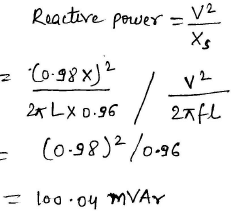Technical Test SSC JE: Electrical Engineering (EE)- 1 - Question 90

The junction capacitance of a p-n junction depends on

Technical Test SSC JE: Electrical Engineering (EE)- 1 - Question 91

In p+n junction diode under reverse bias, the magnitude of electric field is maximum at

Detailed Solution for Technical Test SSC JE: Electrical Engineering (EE)- 1 - Question 91

Electric field is always maximum at the junction

Technical Test SSC JE: Electrical Engineering (EE)- 1 - Question 92

How can the channel width in a junction field effect transistor be controlled?

Technical Test SSC JE: Electrical Engineering (EE)- 1 - Question 93

A Zener diode works on the principle of

Detailed Solution for Technical Test SSC JE: Electrical Engineering (EE)- 1 - Question 93

Due to zener effect in reverse bias under high electric field strength, electron quantum tunneling occurs. It’s a mechanical effect in which a tunneling current occurs through a barrier. They usually cannot move through that barrier.

Technical Test SSC JE: Electrical Engineering (EE)- 1 - Question 94

As compared to full wave rectifier using two diodes, the four diode bridge rectifier has the dominant advantage of

Technical Test SSC JE: Electrical Engineering (EE)- 1 - Question 95

In a transistor, the forward bias across the base emitter junction is kept constant and the reverse bias across the collector base junction is increased. Neglecting, the leakage across the collector base junction & the depletion region generation current, the base current will

Technical Test SSC JE: Electrical Engineering (EE)- 1 - Question 96

Thermal runaway in a transistor biased in active region is due to

Technical Test SSC JE: Electrical Engineering (EE)- 1 - Question 97

The photo electric current in amperes per watt of incident light depend on

Technical Test SSC JE: Electrical Engineering (EE)- 1 - Question 98

In PNP transistor, with normal bias, the emitter junction is

Technical Test SSC JE: Electrical Engineering (EE)- 1 - Question 99

A transistor will operate in inverted region when

Detailed Solution for Technical Test SSC JE: Electrical Engineering (EE)- 1 - Question 99

Forward-active (or simply active)

The base–emitter junction is forward biased and the base–collector junction is reverse biased. Most bipolar transistors are designed to afford the greatest common-emitter current gain, βF, in forward-active mode. If this is the case, the collector–emitter current is approximately proportional to the base current, but many times larger, for small base current variations

Reverse-active (or inverse-active or inverted)

By reversing the biasing conditions of the forward-active region, a bipolar transistor goes into reverse-active mode. In this mode, the emitter and collector regions switch roles. Because most BJTs are designed to maximize current gain in forward-active mode, the βF in inverted mode is several times smaller (2–3 times for the ordinary germanium transistor). This transistor mode is seldom used, usually being considered only for failsafe conditions and some types of bipolar logic. The reverse bias breakdown voltage to the base may be an order of magnitude lower in this region.

Technical Test SSC JE: Electrical Engineering (EE)- 1 - Question 100

In a NPN transistor if the emitter junction is reverse biased and collector junction is reverse biased, the transistor will operate in

## Mock Test Series for SSC JE Electrical Engineering

2 videos|1 docs|55 tests
 Use Code STAYHOME200 and get INR 200 additional OFF Use Coupon Code
Information about Technical Test SSC JE: Electrical Engineering (EE)- 1 Page
In this test you can find the Exam questions for Technical Test SSC JE: Electrical Engineering (EE)- 1 solved & explained in the simplest way possible. Besides giving Questions and answers for Technical Test SSC JE: Electrical Engineering (EE)- 1, EduRev gives you an ample number of Online tests for practice

## Mock Test Series for SSC JE Electrical Engineering

2 videos|1 docs|55 tests